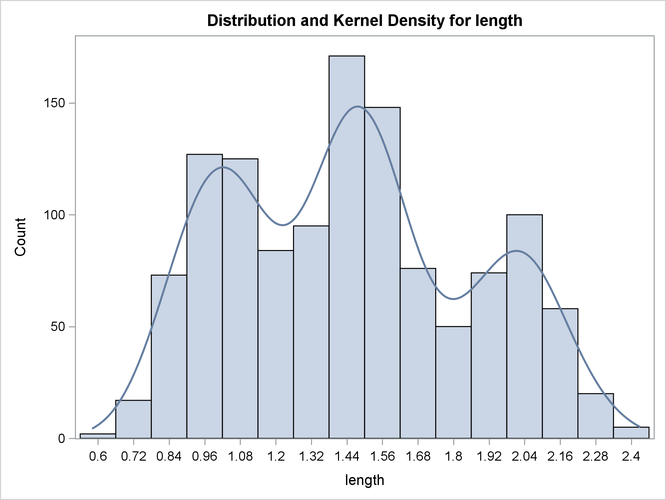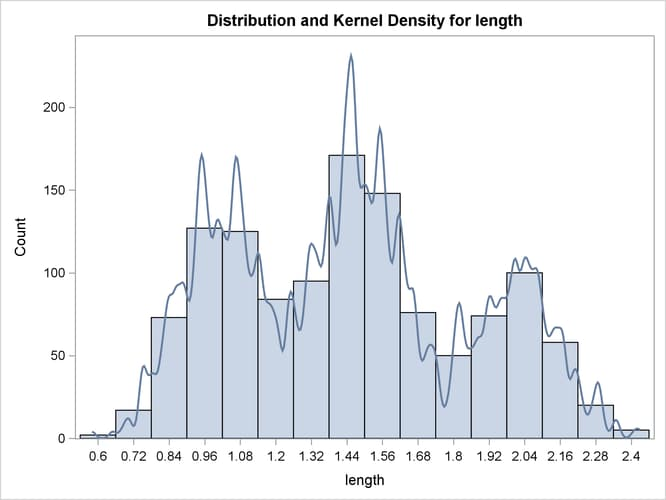# The KDE Procedure

### Example 66.2 Changing the Bandwidth

Continuing with Example 66.1, you can specify different bandwidth multipliers that determine the smoothness of the kernel density estimate. The following statements show kernel density estimates for the variable `length` by specifying two different bandwidth multipliers with the BWM= option:

```proc kde data=channel;
univar length(bwm=2) length(bwm=0.25);
run;
ods graphics off;
```

Output 66.2.1 shows an oversmoothed estimate because the bandwidth multiplier is 2. Output 66.2.2 is created by specifying BWM=0.25, so it is an undersmoothed estimate.

Output 66.2.1: Histogram with Oversmoothed Kernel Density EstimateOutput 66.2.2: Histogram with Undersmoothed Kernel Density Estimate